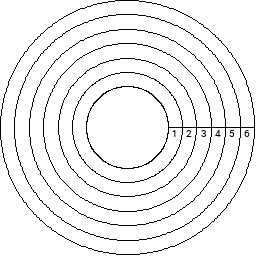2014 ACM ICPC South Central USA Regional Programming Contest

# Runners

Several runners are running on a track which consists of 6 circular lanes. The innermost lane is 400m (meters) long; after running 400m, the runner is back at their starting position. Each outer lane progressively grows by 6m; the 2nd innermost lane is 406m long, and the outermost lane is 430m long. All runners start next to each other and run in the same direction around the track. Each runner runs at a constant rate for the entire race.Determine how often all of the runners are next to each other. For this problem, imagine that each runner is a single point. Runners are considered to be "next to each other" if a line segment can be drawn from the center of the track to the outside of the track going through all of the runners.

# Input

The first line of input contains the number of test cases, T, (1 <= T <= 50). The first line of each test case contains the number of runners, R, (2 <= R <= 6). The next R lines each contain a runner's speed, starting with the runner in the innermost track and moving outward. The speeds are in meters per second and are all within the range 1 <= S <= 11. Each speed may contain a decimal point and up to 2 digits after the decimal point.

# Output

For each test case, print a single line containing the frequency in seconds at which all runners are exactly next to each other, rounded to the nearest integer. If the runners are never all next to each other (other than at the start of the race) or are always all next to each other, print "Unable to solve" instead.

```1
2
4
8
```

```103
```